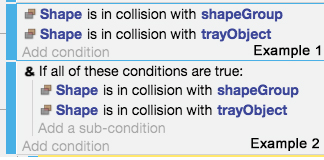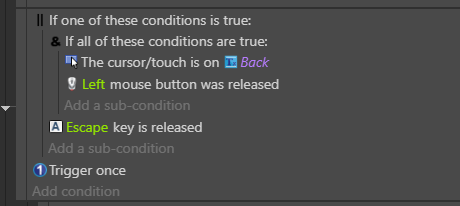# To AND or not to AND, that is the question

Getting near to finalizing my first GDevelop project and I noticed that I have been inconsistant in how I am coding the event conditions. Is there a technical preference for using Example 1 vs Example 2 below?I realize that this may seem a bit silly, but one never knows how a code generator will react to the same statement coded different ways. I doubt there will be a performance issue, but it helps to have coding guidelines and best practices to work by.

Thanks,
Art.

1 Like

You can actually take a look if you export to folder.
The code GD generate is too long to pass it here but in a nutshell the code of the second event is longer and check 3 conditions not only 2.

This is how the code looks like, this is not real actual code this is just to demonstrate.

The code of the first event

``````condition1 = false
condition2 = false

condition1 = Shape.Collision(shapeGroup) //return true on collision
if(condition1 == true)
{
condition2 = Shape.Collision(trayObject) //return true on collision
}
if(condition2 == true)
{
//execute action
}
``````

The code of the second event

``````condition0 = false //this is the AND operation
condition1 = false
condition2 = false

condition1 = Shape.Collision(shapeGroup) //return true on collision
if(condition1 == true)
{
condition2 = Shape.Collision(trayObject) //return true on collision
}
if(condition2 == true)
{
condition0 = true && condition1 && condition2 //test again if both condition is true and return true if it is
}
if(condition0 == true)
{
//execute action
}
``````

So as you can see technically the second event is more complex and you do more conditional operations to get to the same conclusion. So it is obviously not practical and if you do it a lot, could hit performance.

3 Likes

I think the condition block is an AND by default, so you only need to use the AND condition in certain cases, when you need to make a separate AND block. An example:Here I check either the escape key, either both the cursor and the left click.

2 Likes

Thanks, ddabrahim - that is exactly the info I was looking for. Maybe a best practices tag needs to be created to help others find info like this.